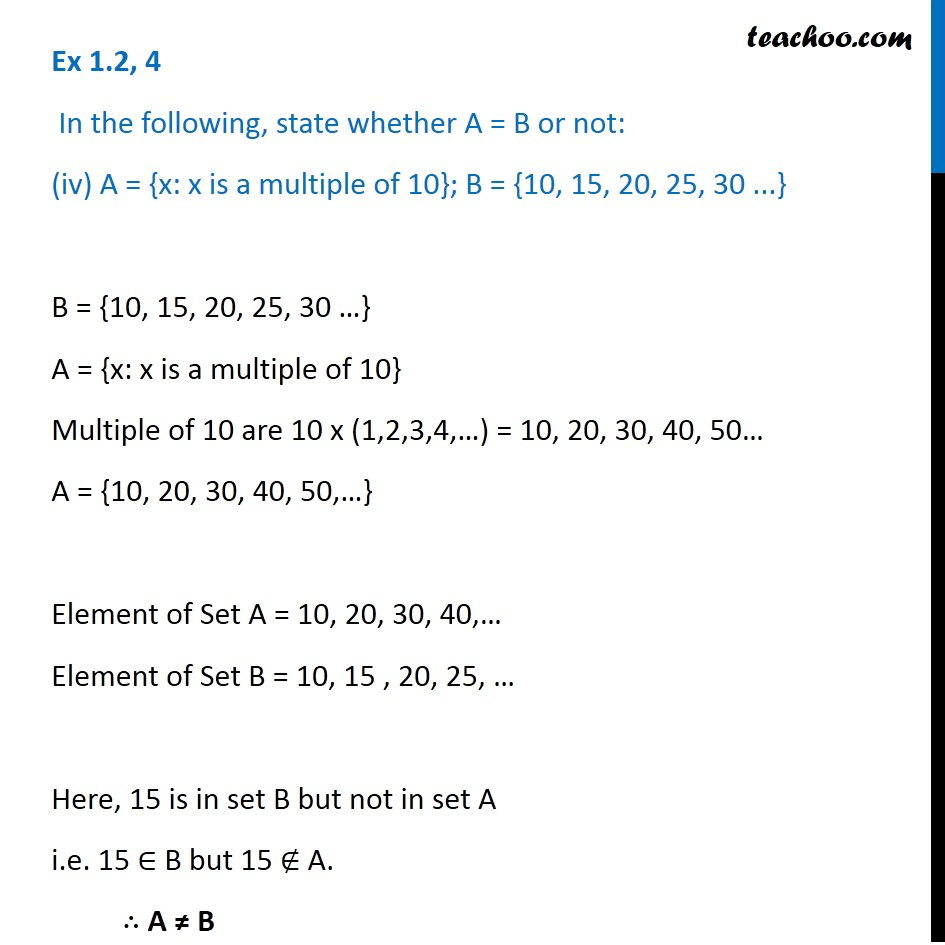1. Chapter 1 Class 11 Sets (Term 1)
2. Serial order wise
3. Ex 1.2

Transcript

Ex 1.2, 4 In the following, state whether A = B or not: (iv) A = {x: x is a multiple of 10}; B = {10, 15, 20, 25, 30 ...} B = {10, 15, 20, 25, 30 …} A = {x: x is a multiple of 10} Multiple of 10 are 10 x (1,2,3,4,…) = 10, 20, 30, 40, 50… A = {10, 20, 30, 40, 50,…} Element of Set A = 10, 20, 30, 40,… Element of Set B = 10, 15 , 20, 25, … Here, 15 is in set B but not in set A i.e. 15 ∈ B but 15 ∉ A. ∴ A ≠ B